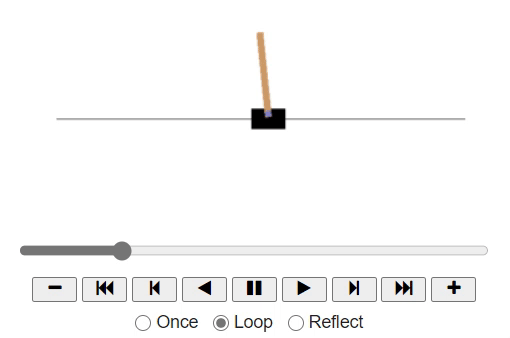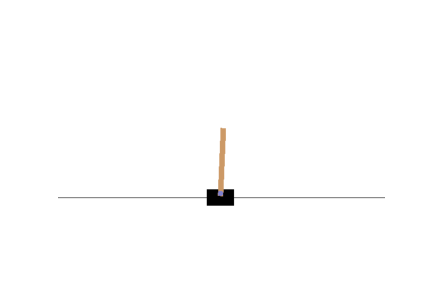# A simple hard-coded policy

• Now that we understand the basic aim to balance the pole upright, how could we do that?

• Well, we need to come up with a policy (or strategy) the agent may follow to achieve the balance at each step. It can use all the past actions and observations to decide what to do.

• As we observe the game, we may naively come to a thought that we need to move the cart to the right if the pole slants towards the right. As the pole tilts towards the left, we might want to push the cart to the left.• Now, let's code the same policy inside the definition `basic_policy`:

INSTRUCTIONS
• Let's hard code our strategy as discussed above, by defining `basic_policy` function:

``````env.seed(42)

def basic_policy(obs):
angle = obs
if angle < 0:
return 0
else:
return 1
``````
• Now let us play 500 episodes of the game, each episode with 200 steps. For each step we call the `basic_policy` to get the `action`, and perform that step with that `action`. Let us calculate the rewards for each episode and finally see how many minimum, maximum, and mean steps that our `basic_policy` is able to keep the pole up:

``````totals = []
for episode in range(500):
episode_rewards = 0
obs = env.reset()
for step in range(200):
action = basic_policy(obs)
obs, reward, done, info = env.step(action)
episode_rewards += reward
if done:
break
totals.append(episode_rewards)
``````
• Now calculate the mean, start deviation, minimum, and maximum number of steps that the pole was upright.

``````print(np.mean(totals), np.std(totals), np.min(totals), np.max(totals))
``````

Well, as expected, this strategy is a bit too basic: the maximum number of steps the agent that kept the pole up is only 68. This environment is considered solved when the agent keeps the poll up for 200 steps.Clearly the system is unstable and after just a few wobbles, the pole ends up too tilted: game over. We will need to be smarter than that! Let us enter the neural network into our game!

No hints are availble for this assesment

Answer is not availble for this assesment

Note - Having trouble with the assessment engine? Follow the steps listed here